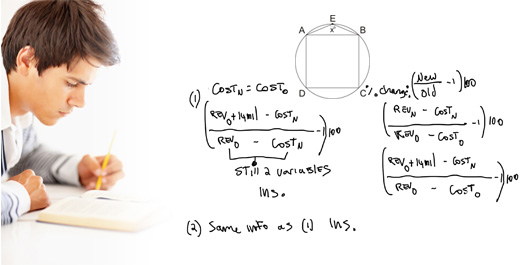Our Address: FZ LLC G 37 & G 34, Block 13, Al Sufouh Road Knowledge Park, Dubai PO Box : 501703

# More Facts on FactorialIn our last article we discussed the way to find number of zeroes in the end of any factorial. For example to find number of zeroes in 22! we just check the number of multiples of 5 in 22!, i.e. 5, 10, 15 and 20 thus 22! ends in 4 zeroes. The same logic can be used in twisting the GMAT questions in many different ways. One interesting question category is as follows:

Q. If N is product of all consecutive integers from 1 to 30, inclusive, what is the greatest integer q for which 10^q is a factor of N?

In this question we are expected to find the maximum power of 10 which divides 30! 10 always ends in zeroes, likewise powers of 10 also, 10^1 = 10 ends in one zero, 10^2 = 100 ends in two zeroes, 10^3 = 1000 ends in 3 zeroes, so on. Thus, the power of 10 represents the number of zeroes in the end. So, the above question can be simply said, find the number of zeroes 30! is ending in. As per our method, lets find the number of multiples of 5 in 30!, i.e. 5, 10, 15, 20, 25 (but 25 have 2 multiples of 5 as 25= 5×5), and 30, so in all we have 7 multiples of 5, thus, 30! ends in 7 zeroes. The maximum power of 10 which divides 30! is 10^7.

Now, at times there are chances we will forget to consider the two multiples of 5 in 25 or say 3 multiples of 5 in 125. So, the best way to find out the maximum power of 5 in any factorial say 30! is, divide 30 by 5, and again divide the quotient by 5, the addition of integer value of quotients gives you the maximum power of 5 which divides 30! Example, 70! ends in how many zeroes. So we go 70/5 = 14, 14/5= 2. Thus, the answer is 14 + 2 = 16.

The same question category can be expanded in following:

Q. If 7^k is a factor of 80!, what is the greatest possible value of k?

So we use the same method: 80/7 = 11

11/7 = 1. Thus, 11+1 = 12. Maximum value for k is 12.

Likewise,

Q. If 15^k is a factor of 90!, what is the greatest possible value of k?

Now, 15 = 3×5. We know that after every three numbers we have a multiple of 3, and every 5 numbers we have a multiple of 5. Thus, we definitely have more 3’s than 5’s. But for one 15 we need one 3 and one 5. So the number of 5’s will decide the number of 15’s in 90!.

Thus, 90/5 = 18. 18/5 = 3. Therefore, 90! has 18+3 = 21 5’s thus maximum value for k is 18.

Q. If N is the product of all positive even integers from 2 to 40. It will have how many trailing zeroes?

Trailing zeroes means, the number N will end in how many zeroes at the end.

N= 2x4x6x….x38x40

We get that N is a product of 20 even numbers, we can take 2 common out of each even number. As 2 is removed from each term, all the rest terms are consecutive natural numbers.

N = 2^20(1x2x3x4x…x19x20)

In order to have trailing zeroes, the number must be divisible by 10. i.e. one 2 and one 5. As discussed earlier, number of 5’s will decide the number of 10’s. Thus, 20/5 = 4, as 4 < 5, it cannot be further divided by 5. Therefore the number N ends in 4 trailing zeroes.